# Zero matrix#### Everything You Need in One Place

Homework problems? Exam preparation? Trying to grasp a concept or just brushing up the basics? Our extensive help & practice library have got you covered.#### Learn and Practice With Ease

Our proven video lessons ease you through problems quickly, and you get tonnes of friendly practice on questions that trip students up on tests and finals.#### Instant and Unlimited Help

Our personalized learning platform enables you to instantly find the exact walkthrough to your specific type of question. Activate unlimited help now!##### Intros
###### Lessons
1. Zero Matrix Overview
##### Examples
###### Lessons
1. Adding, Subtracting and Scalar Multiplication of Zero Matrix
Calculate the following:
1.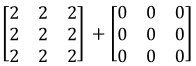2.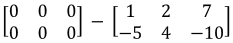3.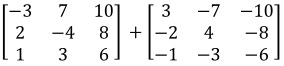4.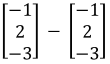5.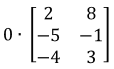6.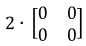2. True or false?
You are given a matrix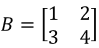and the zero matrix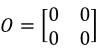. Is the following true? If it is false, then fix the matrix equation.
1. $B+O=B$
2. $O-B=B$
3. $B-B=O$
4. $O+O=B$
5. $0 \cdot B=O$
6. $B \cdot 0=O$
###### Topic Notes
In this section, we will learn about zero matrices. Zero matrices are matrices where all the entries are zero. We see what happens if we add and subtract matrices with zero matrices. Then we will take a look some cases which involves multiplying a 0 scalar with matrix, and multiplying a scalar with a zero matrix. Lastly, we will answer some true or false questions that will help us understand the property of zero matrices.

### What is a zero matrix?

Recall from our lesson on notation of matrices that a matrix is an ordered list of numbers put into a rectangular bracket. For a zero matrix things simplify since you really don't have to worry about the numbers contained in the rectangular array of this notation, just as the name says it, there is only one number that can be contained inside these matrices as all of its entries.

Thus, the zero matrix is a matrix with any dimensions in which all of its element entries are zeros. Mathematically speaking, a zero matrix can be represented by the expression: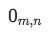Equation 1: Mathematical expression for a zero matrix of dimensions mxn

Where m represents the number of rows and n the number of columns, contained in the matrix. Therefore, if we are to write zero matrices of different sizes, we just have to define m and n in each case and fill all of the entries inside the matrices' brackets with zeros.

Examples of zero matrices can be seen below:

From the zero matrix notation examples above, notice that these matrices can come in any size and dimension combination, and they are not necessarily square matrices. Thus, you can have a zero matrix with any amount of rows or columns, but remember, for any given size it is possible to obtain only one zero matrix (which makes sense, since there is only one way to have all zeros as entries in a matrix of a particular size or dimension combination).

Do not confuse the zero matrix with something people may call a "zero diagonal matrix". Such zero diagonal matrix usually refers to a hollow matrix, where all the diagonal elements inside of it are zero, while the rest of its elements can be any number. The similarity between a regular zero matrix and a hollow matrix comes from their trace (the addition of the elements on their diagonals) since both have all zero elements to be added to produce a trace equal to zero. Thus, both of these two types of matrices are what we call a zero trace matrix.

Important notes about the zero matrix

Once we have learned the zero matrix definition, let us talk about a few of this matrix's special characteristics.

• What is the rank of a zero matrix?
Remember that the rank of a matrix corresponds to the maximum amount of linearly independent columns inside the matrix. We can define the rank of a matrix by computing its row echelon form and then counting the left non-zero rows, the purpose of which is to find the dimension of the vector space for the matrix in question.
So, if we talk about a solvable system of linear equations transformed into a matrix notation, finding the rank of such matrix allows us to see the maximum amount of independent variables, and thus dimension planes for which the system could be graphically represented.
How can we obtain this for a zero matrix then? For that we first need to ask ourselves, are the vectors inside a zero matrix linearly independent from one another? Not really, they are all the same, and they are all zero vectors. Do they happen to represent any dimension plane then? No. Can you actually row reduce it into a row echelon form? No. Thus, if you think about it, a zero matrix contains zero amount of linearly independent columns, and zero amount of non-zero rows, and so our final conclusion is that the rank of zero matrix must be zero.
If you think this idea more in depth, you will realize that any non zero matrix cannot have a rank smaller than one, in other words, in order for any matrix to have a rank of zero, it must contain all zero elements inside, and so, our conclusion is that only zero matrices have a rank of zero.
• Is the zero matrix invertible?
For practical purposes we will leave the complete explanation on how to know if a matrix is invertible or not, and how to invert those which are for our next lessons talking about the 2x2 invertible matrix. For now, we will plainly say that a zero matrix is not invertible.
There are a few rules which can prove this, such as its determinant being zero and if a square matrix, its rank smaller than its dimensions. Again, we will talk a bit more about this on our next lessons about inverting matrices. But let us think about this idea for a minute: If we have mentioned before that for any matrix of a certain size or dimensions, there exists only one configuration in which all of its entries are zero, therefore, there cannot be other way in which you can rearrange the zeros to obtain an inverse matrix which would have the same dimensions. All the entries being the same, the matrix would be exactly the same, there is not an "inverse" or "opposite" from that.
• Is the zero matrix diagonalizable?
We are still a bit away from our lesson in diagonalization, but for now we can say that yes, a zero matrix is diagonalizable since its zero elements can easily contain linearly independent eigenvectors. More on diagonalization in later lessons.

### Null space of zero matrix

Since the zero matrix is a small and concrete concept in itself which can be used through many of our lessons in linear algebra, we are now forced once more to enter into the topic of a later lesson: the null space of a matrix.

Let us keep it simple once more and say that for a vector to be part of the null space of a matrix, the multiplication of such matrix times the mentioned vector should result in a zero vector, thus producing a "null" result.
If our matrix in question is a matrix called A which is being multiplied by the vector u, we say that u is in the null space of A if the following condition is met: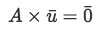Equation 3: Condition for vector u to be part of the null space of A

If we take what we know from our lessons about representing a linear system as a matrix and the matrix equation Ax=b, we can observe that in such multiplication, the vector being multiplied actually represents the set of solutions given by a homogeneous system.

Now, how can this be applied to the zero matrix?
Well, any zero matrix multiplied to a vector will have as a result a zero vector. That is, if the dimensions of the matrix and the vector follow the rules of matrix multiplication, in other words, if the multiplication can be defined, then the result will certainly be a zero vector.
The reason for that is that given that a zero matrix contains only zero elements, any entry multiplied to any element in the vector will result in a zero component which will be part of the resulting vector. So, the condition for null space is met and this takes us to something important we have not mentioned so far: A zero matrix is what we call a null matrix, and this can be clearly seen when following the process described above, since, no matter what vector is multiplied to it, the result will always contain zero elements only.

### Addition, subtraction and scalar multiplication of zero matrix

On this section we will focus on showing examples of operations with either zero matrices inside being operated on, or problems resulting in zero matrix solutions. For that let us jump directly into example exercises:

Example 1

We start with an addition containing a zero matrix. This happens to be quite a simple operation so let us start by having the addition looking like:

To solve this, we just add each corresponding element entries on both matrices to produce the resulting matrix (which has the same dimensions as the ones it comes from). And so, the result looks like:
This first example problem shows us an important property of the zero matrix: When a zero matrix is either added or subtracted from another matrix with the same dimensions, that matrix remains unchanged and is equal to the result of the operation.
Example 2
To proceed with our next example, we work on a subtraction of matrices where a zero matrix is being subtracted to another matrix of equal size.
The operation follows the same principles as the addition in example 1. Thus, solving this operation we obtain:
As we had mentioned in our lesson about adding and subtracting matrices, although matrix addition is commutative (you can change the order of the matrices and the result won't change), matrix subtraction is not, and it is clearly visible on this example.
If you were to have had the zero matrix on the right side of the minus sign on equation 6, then the result would have been equal to the other matrix involved in the operation. But since the zero matrix was first, the result happens to be the negative of the non-zero matrix from the operation.
Example 3
For this example, we have the addition of the next two following matrices:
Notice something in particular from the matrices above? They are the negative matrix of one another, or in other words, if you take the first matrix and multiply it by negative one, you would obtain the second matrix. Therefore, this particular operation is equivalent to subtract a matrix from itself. To show this, let us define the first matrix as A:
Then we write down the equivalent operation we have explained a moment ago:
Notice the scalar multiplication of minus one times A has been simplified to just write it down as a subtraction of the two matrices, which by now, are both A, and so what we have in equation 10 could be just written as: A - A which has obviously a result of zero. But since we are not talking about just numbers here, but matrices, the zero result would have to be an array of the same dimensions as A, and so:
Notice that the subindexes in the right hand side of the equation denote the dimensions of the zero matrix, which mean that the resulting zero matrix must have the "m of A" (the same amount of rows as A) and the "N of A" (the same amount of columns as A). Let us obtain the result in two different ways: the original matrix addition shown in equation 8 and the matrix subtraction found at the end of equation 10, to show how we will obtain the same result: a zero matrix, to prove equation 11.
The conclusion from this problem is that, whenever you subtract a matrix from itself, you will obtain a zero matrix with the same dimensions as your original matrices. Example 4
On this example we will see the subtraction of two equal matrices which happen to be column vectors.
Here, the principle explained during the past exercise is used again: When subtracting two equal matrices (which in this case happen to be two column vectors since the matrices are composed of only one column each), the result is a zero matrix the same size as the original ones:
Example 5
Compute the following scalar multiplication of a matrix:
On this particular case it should be clear the result will be zero since anything you multiply by zero will result in a zero. The interesting part here comes from the fact that you are multiplying a matrix, and so, each element will be multiplied by the scalar outside in this case zero, and what will happen is that, instead of obtaining simply a zero as a result, this multiplication will produce a matrix in which all of its elements are zero, and so, the result is a zero matrix: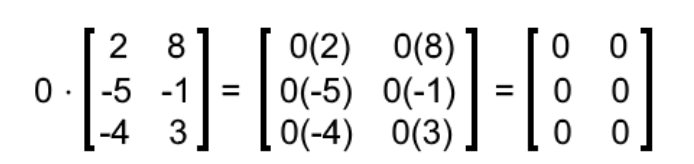Equation 16: Result of scalar multiplication of a matrix by zero
Which can also be written as:
Example 6
Compute the next scalar multiplication containing a zero matrix
Just as with past problems, we can intuitively write down the answer as a zero matrix, since every element in the matrix is a zero, it doesn't matter if you multiply any other scalar to them, the result will always be zero in each case. To expand the operation, here is how it goes: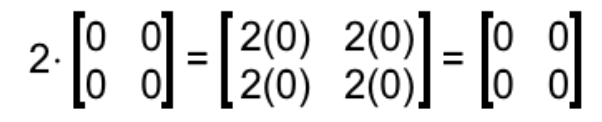Equation 19: Result of the scalar multiplication of a zero matrix
Example 7
Let us change the mode of our problems, now you are given the matrices shown below:
With that in mind, are the following matrix equations true? If not, correct them.
1. B + 0 = B
This case corresponds to what we saw in example 1: Having two matrices with the same dimensions, one of them a zero matrix and the other a non-zero matrix, when you add them together the result is equal to the non-zero matrix since the zero matrix does not contribute anything while adding each corresponding element on the two matrices involved in the operation. Therefore, this expression is CORRECT.
2. 0 - B = B
For this case, we can take a look at example 2 and realize this expression is INCORRECT. When subtracting a matrix from a zero matrix of the same dimensions, the result is equal to the negative of the non-zero matrix.
Therefore, the correct expression would be 0 - B = -B
3. B - B = 0
This expression is CORRECT and corresponds to what we saw in examples 3 and 4: If you subtract a matrix by itself, it results in a entry by entry subtraction of a number by itself, and thus a resulting matrix in which all of its entry elements will be equal to zero (the zero matrix 0).
4. 0 + 0 = B
The expression above is INCORRECT. When adding a zero plus a zero, the result is always a zero. This is the case for each element of the resulting matrix when adding a zero matrix plus another equal zero matrix, the result will be an equal zero matrix. Thus, the correct expression is: 0 + 0 = 0.
5. 0 $\cdot$ B = 0
This expression is CORRECT. Every element by element multiplication result from this operation will result in a zero, producing a matrix with elements all zero, thus the zero matrix 0.
6. B $\cdot$ 0 = 0
Just as in case e) this expression is CORRECT since every corresponding element from the non-zero matrix will be multiplied by a zero from the zero matrix.
Cases e) and f) bring about an important conclusion: matrix multiplication is not commutative unless one of the two matrices is a zero matrix. No matter in which order you multiply the elements of each matrix, one of them has all zero elements producing multiplications which all result in a zero.
As mentioned before, the zero matrix happens to be a very concrete concept so there is truly not much more to say about it for this lesson, still, that doesn't mean it will not be used in multiple areas of linear algebra. Just as the number zero in mathematics, the zero matrix provides us a representation of null space which we can still caracterize, in other words, it may contain null elements but its qualities remain there to be used at our convenience with other matrices.
To finalize our lesson, we will just provide two extra links in case you want to visit them and look at how they define the zero matrix and provide a simple example of an addition with a zero matrix. That is it for today, see you in our next lesson!
Note
A zero matrix is a matrix (usually called O) where all the entries are zero. For example,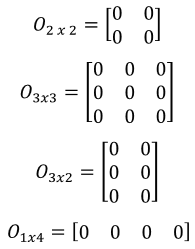are all zero matrices.